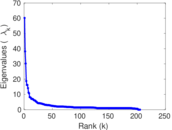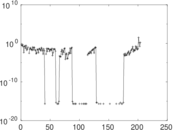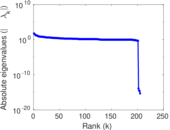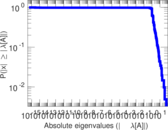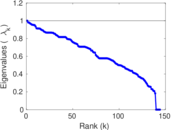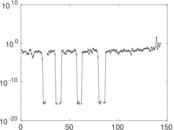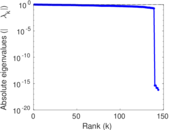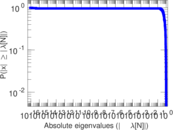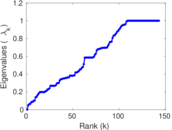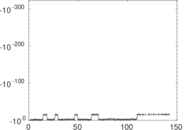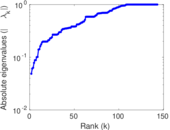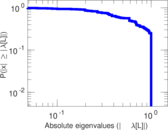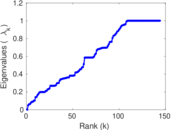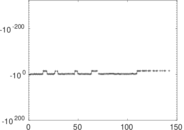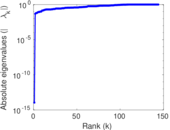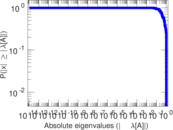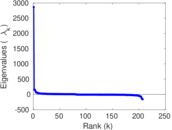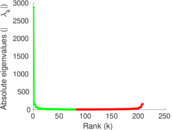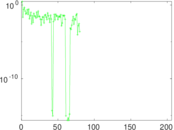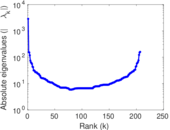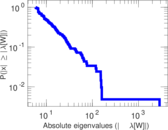# Wikiquote edits (li)

This is the bipartite edit network of the Limburgish Wikiquote. It contains users and pages from the Limburgish Wikiquote, connected by edit events. Each edge represents an edit. The dataset includes the timestamp of each edit.

 Code `qli` Internal name `edit-liwikiquote` Name Wikiquote edits (li) Data source http://dumps.wikimedia.org/ AvailabilityDataset is available for download Consistency checkDataset passed all tests Category Authorship network Dataset timestamp 2017-10-20 Node meaning User, article Edge meaning Edit Network formatBipartite, undirected Edge typeUnweighted, multiple edges Temporal dataEdges are annotated with timestamps

## Statistics

 Size n = 2,869 Left size n1 = 207 Right size n2 = 2,662 Volume m = 5,040 Unique edge count m̿ = 4,336 Wedge count s = 2,367,508 Claw count z = 1,532,583,439 Cross count x = 791,087,647,767 Square count q = 201,309 4-Tour count T4 = 11,097,804 Maximum degree dmax = 2,369 Maximum left degree d1max = 2,369 Maximum right degree d2max = 38 Average degree d = 3.513 42 Average left degree d1 = 24.347 8 Average right degree d2 = 1.893 31 Fill p = 0.007 868 84 Average edge multiplicity m̃ = 1.162 36 Size of LCC N = 2,593 Diameter δ = 12 50-Percentile effective diameter δ0.5 = 1.863 38 90-Percentile effective diameter δ0.9 = 3.910 50 Median distance δM = 2 Mean distance δm = 2.946 71 Gini coefficient G = 0.673 728 Balanced inequality ratio P = 0.236 012 Left balanced inequality ratio P1 = 0.100 992 Right balanced inequality ratio P2 = 0.343 254 Relative edge distribution entropy Her = 0.724 556 Power law exponent γ = 3.784 58 Tail power law exponent γt = 3.141 00 Tail power law exponent with p γ3 = 3.141 00 p-value p = 0.000 00 Left tail power law exponent with p γ3,1 = 1.721 00 Left p-value p1 = 0.061 000 0 Right tail power law exponent with p γ3,2 = 6.581 00 Right p-value p2 = 0.465 000 Degree assortativity ρ = −0.415 790 Degree assortativity p-value pρ = 6.821 61 × 10−181 Spectral norm α = 60.168 0 Algebraic connectivity a = 0.048 535 4 Spectral separation |λ1[A] / λ2[A]| = 1.574 13 Controllability C = 2,447 Relative controllability Cr = 0.858 898

## Plots

### Fruchterman–Reingold graph drawing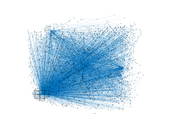### Degree distribution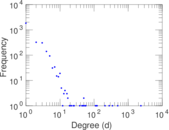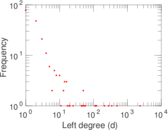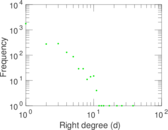### Cumulative degree distribution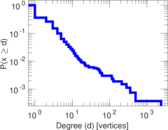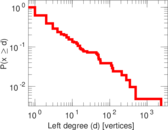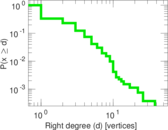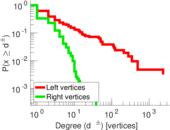### Lorenz curve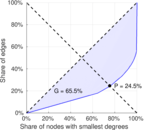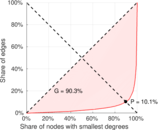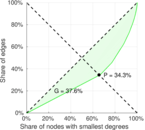### Spectral distribution of the adjacency matrix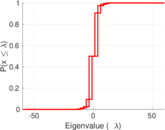### Spectral distribution of the normalized adjacency matrix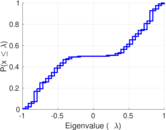### Spectral distribution of the Laplacian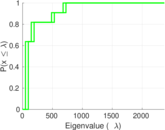### Spectral graph drawing based on the adjacency matrix### Spectral graph drawing based on the Laplacian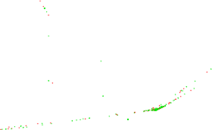### Spectral graph drawing based on the normalized adjacency matrix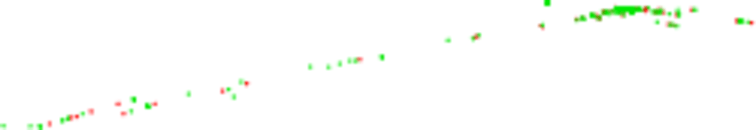### Degree assortativity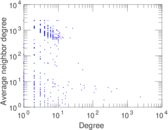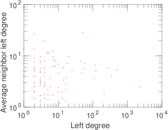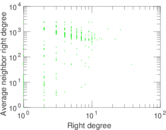### Zipf plot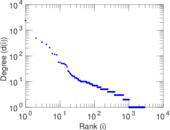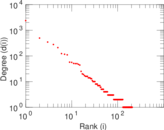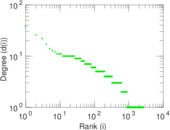### Hop distribution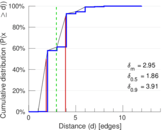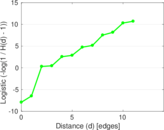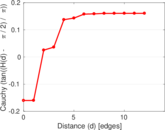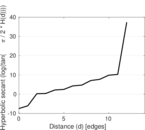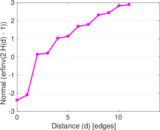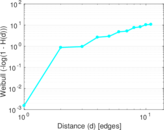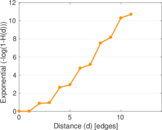### Double Laplacian graph drawing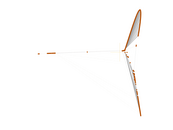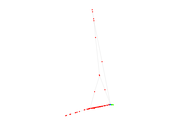### Delaunay graph drawing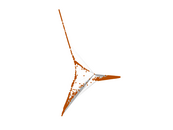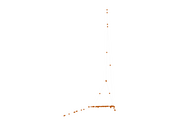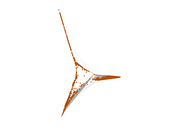### Edge weight/multiplicity distribution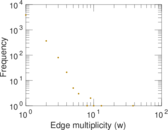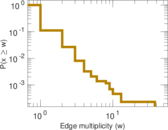### Temporal distribution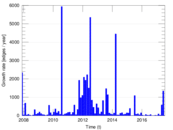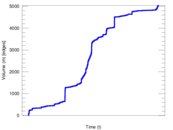### Temporal hop distribution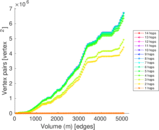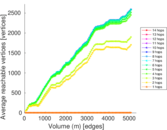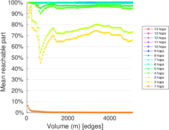### Diameter/density evolution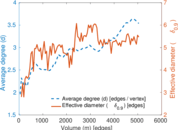### Matrix decompositions plots